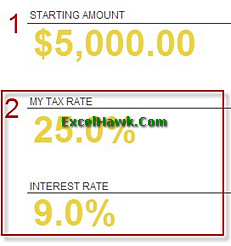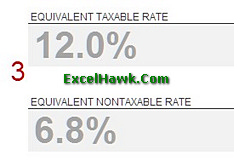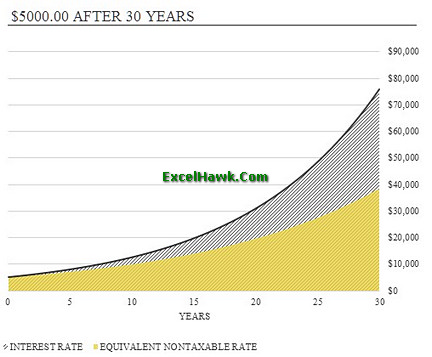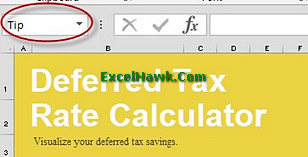# Microsoft Excel Deferred Tax Calculator Template Tutorial

Deferred Tax Calculator is an excel template to calculating the deffered tax of the accounting accounts, whether it is assets or income account. In accounting, deferred tax meaning that a liability account on balance sheet that resulted because of the temporary differences between one of the account in accounting (could be asset, income, or etc) with the tax carrying the values. The deffered tax can be anticipated and enacted by income tax rate and estimated taxe payables for that year. One of the deferred tax example is depreciation. Calculating the deffered tax may be complicated since it has some percentage rates and method to calculate. This is how deferred tax calculation template can help. Upon opening the deferred tax calculation worksheet, it will contain only one application sheet.

HOW TO USE

To use the deferred tax calculator, follow the instructions or you can see the example at the picture below:1. First, input the account value at the Starting Amount field.

2. Fill the My Tax Rate field and Interest Rate field. Both fields are your own estimation over the asset tax rate.

3. The deferred tax calculator will automatically calculate the Equivalent Taxable Rate field and Equivalent Nontaxable Rate field. You can't change the field value since it is auto-generated field.

4. On the left, the deferred tax calculator will show the result of calculation in chart for the next 30 years. If you don't want to set the time that long, you can set the chart into lower year.

You can change the chart style into bar chart or line chart.Notes:

-My Tax Rate: It is the percentage of tax rate charged for an accounting accounts.
-Interest Rate: It is the amount charged by the lender to borrower for the use of asset. Typically expressed in yearly percentage.
-Equivalent Taxable Rate: the return required by taxable investment to make it equal to the return on tax-exempt investment.
-Equivalent Non-Taxable Rate: It is almost same like the Equivalent Taxable Rate, only differ in the usage of tax rate.

You can print the result and editing the charts style. Before you print the deferred tax calculator with the result, go to small drop down menu on the header (marked in pictures below) to check the print area.You can modify the deferred tax calculation example excel for editing the calculation formula or adding a sheet for calculation record. However, it is not really recommended since the template main purpose is to be deferred tax calculator program. If you want to use it with details record, you can collaborate or integrate the calculator template with another software.

TEMPLATE SUMMARY

The free deferred tax calculation in excel can be downloaded at excelhawk.com. You can use it for personal use (asset) or for small to large company regardless of the business industry. You can print the deferred calculator result as a report too. If you have trouble in accounting calculation especially in deferred accounts, you can count on the deferred tax calculator template.